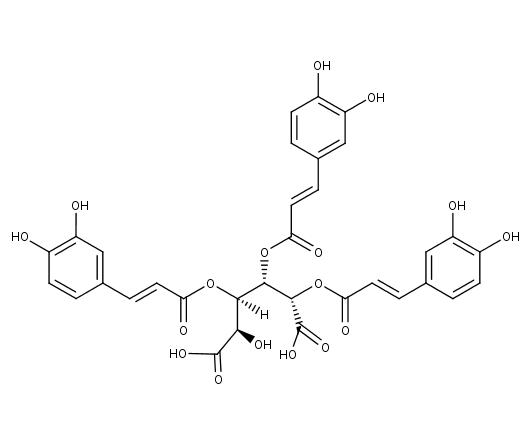Leontopodic acid B analytical standard provided with w/w absolute assay, to be used for quantitative titration.
Synonym
• 3,4,5-Tri-(E)-caffeoyl-D-glucaric acid
280.00 \$
280.00 \$

## identification

6026 S
C₃₃H₂₈O₁₇
933063-22-0
696.58
3,4,5-Tri-(E)-caffeoyl-D-glucaric acid
[H][C@@](OC(=O)\C=C\C1=CC(O)=C(O)C=C1)([C@@H](O)C(O)=O)...
[H][C@@](OC(=O)\C=C\C1=CC(O)=C(O)C=C1)([C@@H](O)C(O)=O)[C@H](OC(=O)\C=C\C1=CC(O)=C(O)C=C1)[C@H](OC(=O)\C=C\C1=CC(O)=C(O)C=C1)C(O)=O

## specification

Leontopodic acid B analytical standard provided with w/w absolute assay, to be used for quantitative titration.
Purity (HPLC) ≥95% (This product is available with HPLC chromatogram)

## plants

Leontopodium alpinum
• Edelweiss (en)

P260 P262

## storage

Store at : <-15°C
Store in dry and dark place.
Ambient temperature